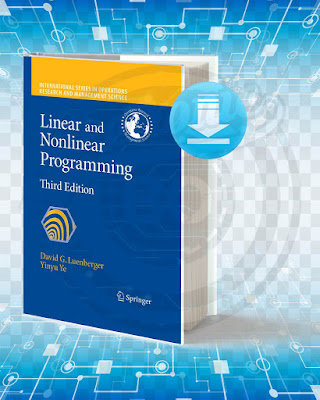## Linear And Nonlinear Programming.

### Picture Of The Book:Linear And Nonlinear Programming

This Book Explains The Central Concepts Of Practical Optimization Techniques, Focusing On Both Modern And Popular Methods. The Main Ideas Is The Relationship Between The Purely Analytical Personality Of The Optimization Problem And The Behavior Of The Algorithms Used To Solve The Problem. And Unrestricted Optimization Theory, Including The Derivation Of Optimization Conditions And Introduction To Basic Algorithms. This Part Of The Book Explores The General Characteristics Of Algorithms And Identifies Different Concepts Of Convergence.

Title: Linear And Nonlinear Programming.
Language: English.
Size: 3.97 Mb.
Pages: 563.
Format: Pdf.
Year: 2008.
Edition: 3.
Author: David G. Luenberger.

### Contents Of The Book:

Chapter 1: Introduction.
Chapter 2: Basic Properties Of Linear Programs.
Chapter 3: The Simplex Method.
Chapter 3: The Simplex Method.
Chapter 4: Duality.
Chapter 5: Interior-Point Methods.
Chapter 6: Transportation And Network Flow Problems.
Chapter 7: Basic Properties Of Solutions And Algorithms.
Chapter 8: Basic Descent Methods.
Chapter 9: Conjugate Direction Methods.
Chapter 10: Quasi-Newton Methods.
Chapter 11: Constrained Minimization Conditions.
Chapter 12: Primal Methods.
Chapter 13: Penalty And Barrier Methods.
Chapter 14: Dual And Cutting Plane Methods.
Chapter 15: Primal-Dual Methods.